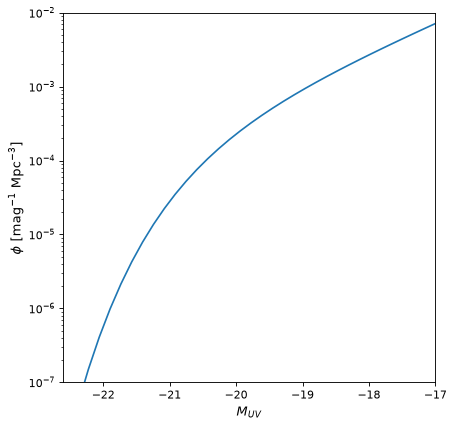# Schechter1D¶

class astropy.modeling.powerlaws.Schechter1D(phi_star=1.0, m_star=- 20.0, alpha=- 1.0, **kwargs)[source]

Schechter luminosity function (Schechter 1976), parameterized in terms of magnitudes.

Parameters
phi_starfloat

The normalization factor in units of number density.

m_starfloat

The characteristic magnitude where the power-law form of the function cuts off. Must not have units.

alphafloat

The power law index, also known as the faint-end slope. Must not have units.

Notes

Model formula (with $$\phi^{*}$$ for phi_star, $$M^{*}$$ for m_star, and $$\alpha$$ for alpha):

$n(M) \ dM = (0.4 \ln 10) \ \phi^{*} \ [{10^{0.4 (M^{*} - M)}}]^{\alpha + 1} \ \exp{[-10^{0.4 (M^{*} - M)}]} \ dM$

phi_star is the normalization factor in units of number density. m_star is the characteristic magnitude where the power-law form of the function cuts off into the exponential form. alpha is the power-law index, defining the faint-end slope of the luminosity function.

References

1

Schechter 1976; ApJ 203, 297 (https://ui.adsabs.harvard.edu/abs/1976ApJ…203..297S/abstract)

2

Luminosity function

Examples

from astropy.modeling.models import Schechter1D
import astropy.units as u
import matplotlib.pyplot as plt
import numpy as np

phi_star = 4.3e-4 * (u.Mpc ** -3)
m_star = -20.26
alpha = -1.98
model = Schechter1D(phi_star, m_star, alpha)
mag = np.linspace(-25, -17)

fig, ax = plt.subplots()
ax.plot(mag, model(mag))
ax.set_yscale('log')
ax.set_xlim(-22.6, -17)
ax.set_ylim(1.e-7, 1.e-2)
ax.set_xlabel('$M_{UV}$')
ax.set_ylabel('$\phi$ [mag$^{-1}$ Mpc$^{-3}]$')


(png, svg, pdf)Attributes Summary

 alpha input_units This property is used to indicate what units or sets of units the evaluate method expects, and returns a dictionary mapping inputs to units (or None if any units are accepted). m_star param_names Names of the parameters that describe models of this type. phi_star

Methods Summary

 evaluate(mag, phi_star, m_star, alpha) Schechter luminosity function model function. fit_deriv(mag, phi_star, m_star, alpha) Schechter luminosity function derivative with respect to parameters.

Attributes Documentation

alpha = Parameter('alpha', value=-1.0)
input_units
m_star = Parameter('m_star', value=-20.0)
param_names = ('phi_star', 'm_star', 'alpha')

Names of the parameters that describe models of this type.

The parameters in this tuple are in the same order they should be passed in when initializing a model of a specific type. Some types of models, such as polynomial models, have a different number of parameters depending on some other property of the model, such as the degree.

When defining a custom model class the value of this attribute is automatically set by the Parameter attributes defined in the class body.

phi_star = Parameter('phi_star', value=1.0)

Methods Documentation

evaluate(mag, phi_star, m_star, alpha)[source]

Schechter luminosity function model function.

fit_deriv(mag, phi_star, m_star, alpha)[source]

Schechter luminosity function derivative with respect to parameters.